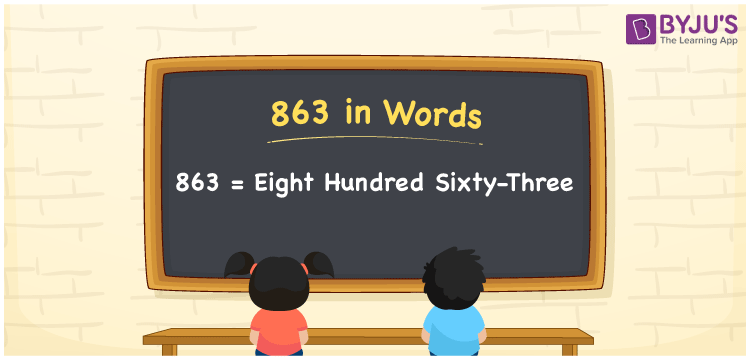# 863 in Words

863 in Words is “Eight hundred sixty-three”. The number 863 is a natural number that lies between 862 and 864. In Mathematics, 863 is said to be a cardinal number, as it is used to express the quantity of objects equivalent to eight hundred sixty-three. Now, let us discuss the conversion of the number 863 into words using the place value system in detail.

 863 in Words: Eight Hundred Sixty-three. Eight Hundred Sixty-three in Numerical Form: 863.

## 863 in English Words## How to Write 863 in Words?

For the three digit number 863, the place values are given below.

 Hundreds Tens Ones 8 6 3

The expanded form of 863 is as follows:

= 8 × Hundred + 6 × Ten + 3 × One

= 8 × 100 + 6 × 10 + 3 × 1

= 800 + 60 + 3

= 863

= Eight hundred sixty-three

Hence, 863 in words is eight hundred sixty-three.

863 in words – Eight hundred sixty-three

Is 863 an odd number? – Yes

Is 863 an even number? – No

Is 863 a perfect square number? – No

Is 863 a perfect cube number? – No

Is 863 a prime number? – Yes

Is 863 a composite number? – No

## Frequently Asked Questions on 863 in Words

### Write 863 in words.

863 in words is eight hundred sixty-three.

### Simplify 800 + 63, and express it in words.

Simplifying 800 + 63, we get 863. Hence, 863 in words is eight hundred sixty-three.

### Is 863 a composite number?

No, 863 is not a composite number.